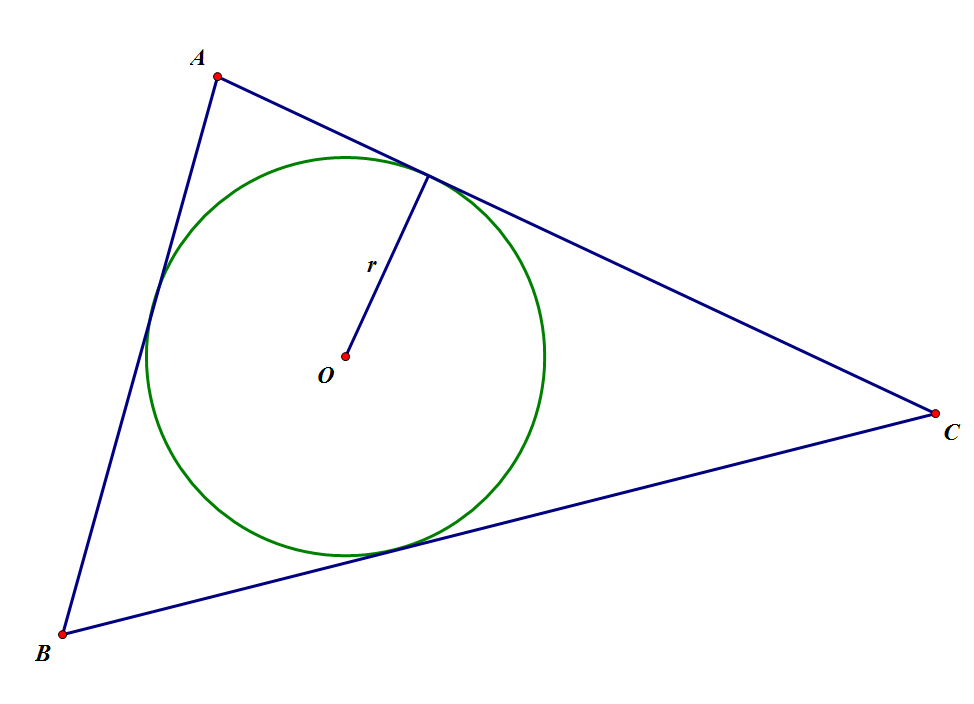# Circle Areas #1: The Basics

Geometry Level 2In the diagram above, Circle O is inscribed into Triangle ABC, where AB = $3$, AC = $4$, and BC = $5$. The area of Circle O can be written as $a\pi$, where $a$ is a constant. What is the value of $a$?

Note: Not to scale

This is part of the set Circles, made by Chris H.

×